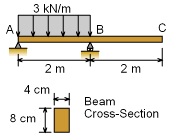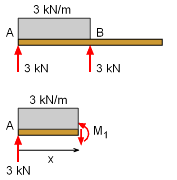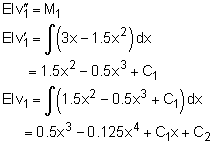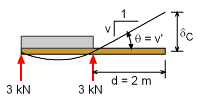Ch 5. Beam Deflections Multimedia Engineering Mechanics Integrationof Moment Integrationof Load Method ofSuperposition IndeterminateBeams
 Chapter 1. Stress/Strain 2. Torsion 3. Beam Shr/Moment 4. Beam Stresses 5. Beam Deflections 6. Beam-Advanced 7. Stress Analysis 8. Strain Analysis 9. Columns Appendix Basic Math Units Basic Equations Sections Material Properties Structural Shapes Beam Equations Search eBooks Dynamics Fluids Math Mechanics Statics Thermodynamics Author(s): Kurt Gramoll ©Kurt GramollMECHANICS - EXAMPLE ExamplePartially Loaded Beam Beams commonly have distributed loads over only sections of the total beam, similar to the beam shown in the diagram. In this case, what is the deflection of the far right end (point C)? Assume the modulus of elasticity, E, is equal to 10 GPa. Solution There are two basic ways to solve this problem using integration. First, the moment in both beam sections, AB and BC, can be determined, and then integrated using two boundary conditions for each span. A second, and easier method, would be to find the rotation angle at B, and then just extrapolate the deflection from B to C. This is possible since there are no loads on the beam section BC and thus there will be no bending. The section BC will rotate, but it remain a straight line. Of course, the rotation at B still needs to be determined by integrating the moment equation, but it is only one equation instead of two equations needed in method 1.Beam Support Reactions Due to symmetry, each of the two supports will carry half the load, giving,      Ay = By = 3(2)/2 = 3 kN The moment equation for the first span, AB, is found by cutting the span at distance x from the left, and summing moments. This gives,      M1 + 3x(x/2) - 3x = 0      M1 = 3x - 1.5x2 kN-m Now that the moment equation is known for the span, it can be integrated once to find the beam rotation, and a second time for beam deflection,The deflection, v1, for the span AB is know at x = 0 and x = 2 m. Using these two boundary conditions, gives      v1(x=0) = 0.5 (0)3 - 0.125 (0)4 + C1 (0) + C2           ==> C2 = 0      v1(x=2) = 0.5 (2)3 - 0.125 (2)4 + C1 (2) + 0           ==> C1 = -1 This give the beam rotation as      EIv' = (1.5x2 - 0.5x3 -1) kN-m2Beam Deflection at Point C The beam moment of inertia, I, is      I = 83(4)/12 = 170.7 cm4 = 170.7e-8 m4 and      EI = (10e9 N/m2)(170.7e-8 m4 ) = 17.07 kN/m2 The beam rotation at B is      v'(x=2) = (6 - 4 -1)/EI                = (1 kN-m2)/(17.07 kn-m2)                = 0.05858 radians The final deflection at C can be determined by noting that that beam rotation at B is also the slope at B. The final deflection is just the angle (or slope) times the distance,       δC = θ d =0.05858 (2 m)          = 0.1172 m

Practice Homework and Test problems now available in the 'Eng Mechanics' mobile app
Includes over 400 problems with complete detailed solutions.
Available now at the Google Play Store and Apple App Store.